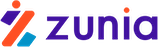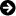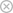Tuyển sinh 2023 dành cho Gen-Z# Analytical Methods: EViews and Panel Data

Chia sẻ: Hgiang Hgiang | Ngày: | Loại File: DOC | Số trang:7352
lượt xem
51

In panel data models (as in single-equation multiple-regression models) we are interested in testing two types of hypotheses: hypotheses about the variances and covariances of the stochastic error terms and hypotheses about the regression coefficients. The general to simple procedure provides a good guide.

Chủ đề:

## Nội dung Text: Analytical Methods: EViews and Panel Data

1. Fulbright Economics Teaching Program First Semester 2002 Analytical Methods EViews and Panel Data EViews POOL objects operate on variables that have special two-part names. The first part is the name of the variable, and the second part of the name is the cross-section identifier that indicates which cross-sectional unit the variable belongs to. It is good practice (though not necessary) to begin cross-section identifiers with an underscore mark to make the full variable names more readable. Example: Suppose we want to work with a panel data set on the USA, Canada, and Mexico. The variables that we want to use are GDP, Population, and Trade Flows. Variable Name First Part: GDP POP TRA Variable Name Second Part (Cross-Section Identifier) _USA _CAN _MEX Variables GDP_USA GDP_CAN GDP_MEX POP_USA POP_CAN POP_MEX TRA_USA TRA_CAN TRA_MEX Thus, there are nine variables in our EViews workfile. It is easy to see that panel data sets can quickly become very large. For example, if we have a panel of 61 Vietnamese provinces for which we have ten-year time series on 8 variables related to agriculture, then our workfile has (61 x 8) = 488 variables. After you have named all of your variables and have got the data into an EViews workfile, you are ready to create the POOL object. You do this by clicking the following sequence: Objects / New Object / Pool A window will open with space for you to list your cross-section identifiers: Eshragh Motahar 1
2. In the POOL object you refer to variables by the first part name and the question mark. Thus, if we type a command using GDP? EViews uses all three GDP series for the USA, Canada, and Mexico. Notice the button PoolGenr. PoolGenr is used to create new variables according to rules that are similar to the rules for ordinary Genr. For example, if we want to create GDP Per Capita for all three countries in our POOL, we would click PoolGenr and then type the equation: GDPPC? = GDP? / POP? For estimation, EViews has one window in which the user specifies the equation and the assumptions regarding the stochastic disturbance term. That window is shown here. We will describe each element of the specification window. Dependent Variable The dependent variable will be typed in according to its name and question mark. For example, you might use GDPPC? Eshragh Motahar 2
3. Sample By default, EViews will use the largest sample possible in each cross-section. An observation will be excluded if any of the explanatory or dependent variables for that cross-section are unavailable in that period. If the box for Balanced Sample is checked, EViews will eliminate an observation if data are unavailable for any cross-section in that period. Common Coefficients In this field you list all explanatory variables that you assume have the same slope coefficient for every cross-sectional unit. Use the format VAR? You may use AR(p) specifications if you want to model autocorrelation. Keep in mind that your panel data set should have a rather long time series dimension in order to get reliable estimators of the autocorrelation coefficients. Cross-Section Specific Coefficients In this window you type the names of all explanatory variables that you assume have different slope coefficient values for different cross-sectional units. Use the format VAR? Intercept Here you specify whether your model has No intercept ... this case is rare. Common intercept ... this case is unusual. Fixed Effects ... the typical specification.1 Random Effects ... this specification is not often used because it requires strong assumptions that are difficult to meet in practice. Weighting Here, weighting refers to "feasible weighted least squares." No weighting ... no equation-specific heteroscedasticity. Cross-section weights ... feasible WLS to correct for equation-specific heteroscedasticity. SUR ...accounts for contemporaneous cross-equation correlation of errors and equation-specific heteroscedasticity. To use this, the time-series dimension must exceed the cross-section dimension (T > N). 1 If you check this option you will see that the EViews output does not report standard errors, t-stats, or p-values for the estimates of the fixed effects. If you are interested in these (especially for conducting Wald test on these coefficients), you can enter C in the Cross section specific coefficients edit field, and estimate a model without a constant; that is, in the Intercept field check None. Eshragh Motahar 3
4. Iterate to Convergence ... causes the program to compute new residuals based on the feasible GLS coefficient estimators, then update the feasible GLS coefficient estimators; compute new residuals based on the new GLS coefficient estimators, then update the feasible GLS coefficient estimators, etc .... Options There is only one option: Whites HCCM can be produced if you do not choose SUR. Hypothesis Testing In panel data models (as in single-equation multiple-regression models) we are interested in testing two types of hypotheses: hypotheses about the variances and covariances of the stochastic error terms and hypotheses about the regression coefficients. The general to simple procedure provides a good guide. Before testing hypotheses about the regression coefficients, it is important to have a good specification of the error covariance matrix so that the test statistics for the regression coefficients are reliable. Testing Hypotheses About The Error Covariance Matrix It is helpful to think about restricted and unrestricted error covariance matrices. An error covariance matrix is a square matrix with the error variances of the individual cross- sectional equations along the diagonal and with the contemporaneous error covariances on the off-diagonal elements. All covariance matrices are symmetric, so if we specify an error covariance matrix for a panel model with five cross-sectional units we have a (5 x 5) matrix with five diagonal units and ten off-diagonal units: σ 12 σ 12 σ 13 σ 14 σ 15 σ2 2 σ 23 σ 24 σ 25 σ 32 σ 34 σ 35 σ4 2 σ 45 σ 52 If we click the button for SUR, EViews will estimate all of these parameters. On the other hand, if we believe that the cross-sectional units do not have any contemporaneous cross-equation error covariances, we would click the button for Cross-Section Weighting and EViews would impose zero restrictions on all of the off-diagonal elements of the matrix. Only the diagonal elements would be estimated: Eshragh Motahar 4
5. σ 12 0 0 0 0 σ 2 2 0 0 0 σ 2 3 0 0 σ 2 4 0 σ 52 The second model involves imposing ten restrictions, compared to the first model. Finally, if we assumed that our stochastic disturbances were free of cross-sectional heteroscedasticity, we click the No Weighting button and EViews would estimate only one diagonal element instead of five: four restrictions would be imposed, compared to the second model. σ2 0 0 0 0 σ 2 0 0 0 σ 2 0 0 σ 2 0 σ2 Testing these restrictions is easily accomplished by means of a test called a likelihood ratio test. EViews output reports a statistic called the Log-Likelihood. This is an estimator of the joint probability of the observed sample, given the point estimates of the parameters. As such, it is a number bounded by zero and one. All of our estimation methods aim to maximize this log-likelihood. In many applications, maximizing the log-likelihood leads to exactly the same estimator as the Least-Squares method does, but the analytical work required is heavier, so we follow the Least-Squares approach. Our interest here is in the extent to which imposing restrictions on the error covariance matrix reduces the log-likelihood statistic. ˆ If we form a ratio of the likelihood of a restricted model LR divided by the likelihood of an ˆ unrestricted model LU , we expect the ratio to be less than 1 because the maximum likelihood subject to a restriction can be no greater than the maximum likelihood of the unrestricted model. ˆ LR l = Define the likelihood ratio: ˆ . Then 0 ≤ l ≤ 1 LU If the restricted model is not significantly different from the unrestricted model we expect the likelihood ratio to be close to 1. The distribution theory of the likelihood ratio is a bit cumbersome. However, it is well known that the distribution of −2 × log(l) is asymptotically Chi-Square, so that in any application with a sufficiently large sample size we can use: Eshragh Motahar 5
6. ( ( )ˆ ˆ( )) −2 × log( l) = −2 × log LR − log LU approx ~ χ q where q is the number of 2 restrictions. Under the null hypothesis we expect −2 × log(l) to be close to zero; we reject the null hypothesis if the realized value of the likelihood ratio statistic exceeds an appropriate critical value or if the p-value of the test is smaller than the pre-selected significance level. Maintained Model While testing hypotheses about restrictions on the error covariance matrix, some specification of the panel data regression model must be maintained. It is recommended that the maintained model be "general" in the sense that we used that term in describing the "general-to-simple" modeling strategy. Testing Restrictions on the Panel Data Model After a sound specification for the error covariance structure has been established, tests associated with the general to simple modeling strategy may be undertaken. These tests may be the usual Wald tests or t-tests on individual coefficients. Keep in mind that when the cross-section weights or SUR methods or any AR(p) specification is used, the results all asymptotically based so that the t-stats are approximately standard normal and the Wald F-stats are approximately Chi-Squared. Unrestricted Model The completely unrestricted model is this one: Yit = αi + β i1 X 1 i t + β i 2 X 2 i t + L + β iK X K i t + ε it In this model, the intercepts and the partial regression coefficients vary across cross-sectional units. If either the no-weighting or cross-sectional weights option is chosen for the error covariance structure, then the results will be exactly the same as applying OLS to the data for each cross sectional unit. If the SUR option is chosen, then efficiency will be enhanced by exploiting the information contained in the cross-equation error covariances. Remember that ( T > N ) is required to use this option. Partially Restricted Model In many panel data sets the time-series dimension is quite short so it is impractical to estimate the model for which all parameters vary across cross-sectional units. In this case the most Eshragh Motahar 6
7. general feasible model is the fixed-effects model: only the intercepts vary across cross-sectional units; the partial regression coefficients are the same for all cross-sectional units. Of course, there are models in which some partial regression coefficients are identical across cross-sectional units while others vary. Restricted Model The most restrictive model is the one in which the intercepts and the partial regression coefficients are identical for all cross-sectional units. Testing model restrictions may be done via the Wald Coefficient test or via the likelihood ratio test. The two methods are asymptotically equivalent, though they may give different results for a particular finite sample. As you move through the general-to-simple modeling strategy it is sensible to re-check the error covariance structure as you impose restrictions on the model's partial regression coefficients and intercepts. Even though you may fail to reject the hypotheses that represent restrictions that you impose, the hypotheses may not be perfectly true, and that may affect the estimators and tests of the error covariances. [Written by Eshragh Motahar based on notes by M. D. Westbrook, and the EViews manual.] Eshragh Motahar 7